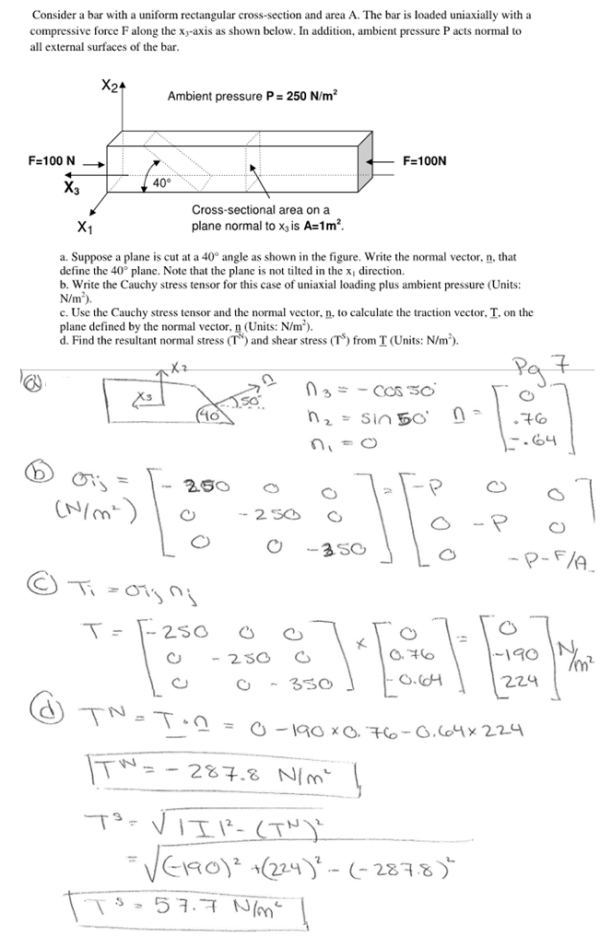Consider a bar with a uniform rectangular cross section and area A. The bar is loaded uniaxially with a compressive force F along the xy-axis as shown below. In addition, ambient pressure P acts normal to all external surfaces of the bar. Suppose a plane is cut at a 40 degree angle as shown in the figure. Write the normal vector, n, that defines the 40 degree plane. Note that the plane is not tilted in the x direction. b) Write the Cauchy stress tensor for this case of uniaxial loading plus ambient pressure. Use the Cauchy stress tensor and the normal vector to calculate the traction vector, T, on the plane defined by the normal vector, n. Find the resultant normal stress and shear stress from T.Consider a bar with a uniform rectangular cross section and area A. The bar is loaded uniaxially with a compressive force F along the xy-axis as shown below. In addition, ambient pressure P acts normal to all external surfaces of the bar. Suppose a plane is cut at a 40 degree angle as shown in the figure. Write the normal vector, n, that defines the 40 degree plane. Note that the plane is not tilted in the x direction. b) Write the Cauchy stress tensor for this case of uniaxial loading plus ambient pressure. Use the Cauchy stress tensor and the normal vector to calculate the traction vector, T, on the plane defined by the normal vector, n. Find the resultant normal stress and shear stress from T.

biomechanics page 1 biomechanics biomechanics biomechanics biomechanics biomechanics biomechanics biomechanics biomechanics page 2